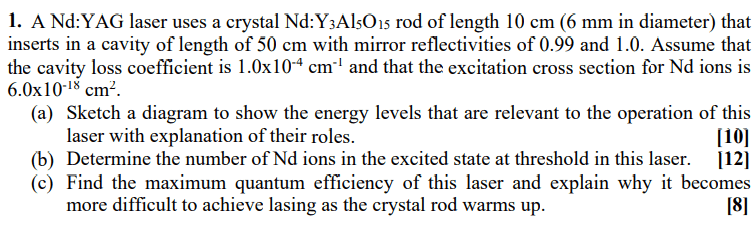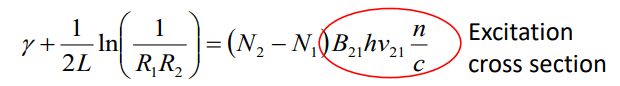# Nd:YAG laser with a crystal Nd:Y3Al5O15 rod -- How many ions are in the excited state?

Homework Statement:
A Nd:YAG laser with a crystal Nd:Y3Al5O15 rod. Determine the number of Nd ions in the excited state at threshold in this laser.
Relevant Equations:
$\gamma+\frac{1}{2L} ln(\frac{1}{R_{1}R_{2})=(N_{1}-N_{2})\sigma}$For part b)Gamma is the cavity loss coefficient, L is the lenght of the rod, R1,2 are the reflectivities. I am not sure how to find the number of ions in the excited state since I don't know the number of ions in the ground state.

•Delta2

•